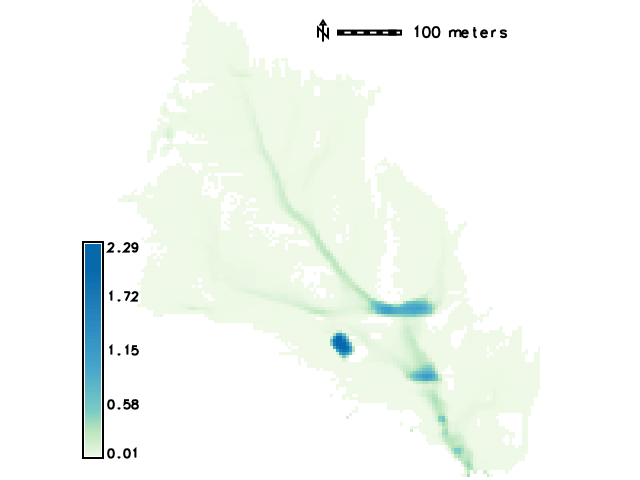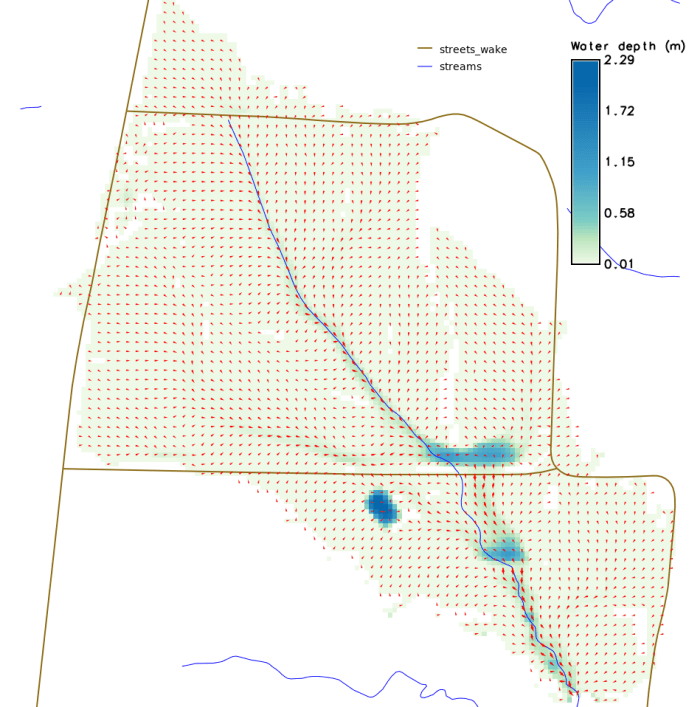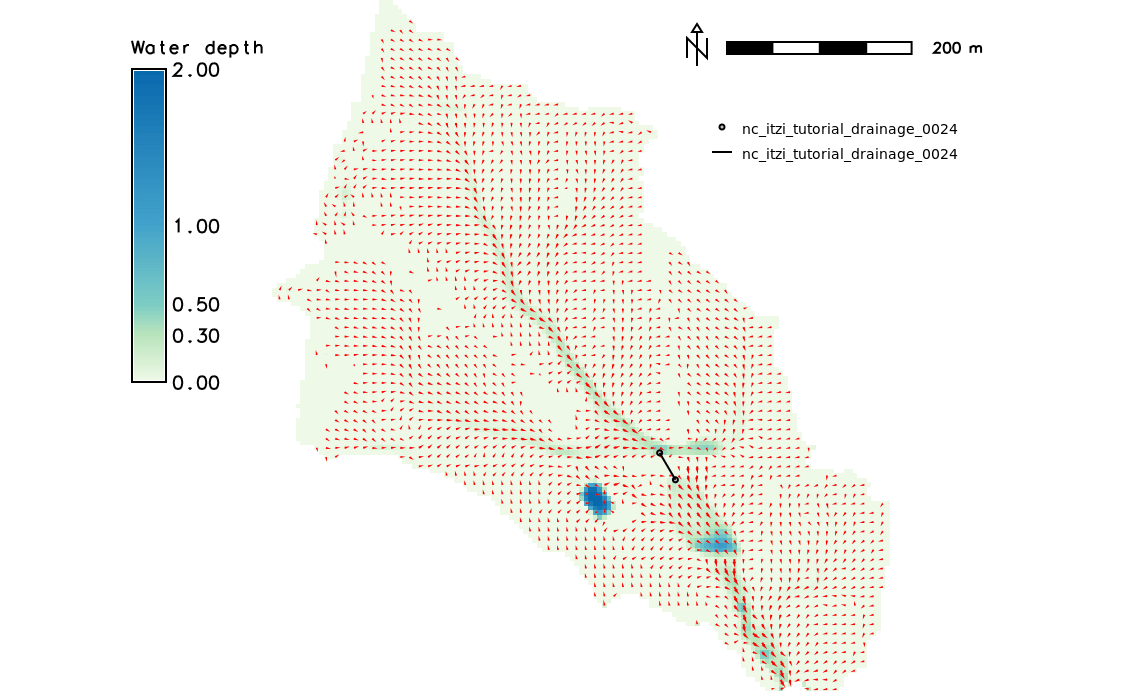# Tutorial¶

This tutorial shows how to run a basic Itzï simulation using freely available dataset.

It assumes that GRASS 7 and Itzï are properly installed on your machine and that you possess a basic knowledge of GRASS.

Then start GRASS in the PERMANENT mapset.

## Surface modelling¶

Fit the lidar elevation raster map and set a resolution of 5m:

$g.region -p raster=elev_lid792_1m@PERMANENT res=5 save=lidar_5m  ### Resample the DEM¶ Please note that this step is not strictly necessary. The Itzï simulation will be carried out in any case on the defined computational region extent and resolution. However the bilinear interpolation smooth the surface, which prevent high slope values that could occur if using the GRASS default nearest-neighbour sampling. $ r.resamp.interp input=elev_lid792_1m@PERMANENT output=elev_lid792_5m


Generate a drainage direction map and then create a watershed raster using the outlet point coordinates:

$r.watershed elevation=elev_lid792_5m drainage=elev_lid792_5m_drainage$ r.water.outlet input=elev_lid792_5m_drainage output=watershed coordinates=638888,220011


Create a raster mask to prevent calculation outside of the watershed:

$r.mask rast=watershed  ### Create boundary condition maps¶ Create a vector map with the watershed outlet point: $ echo '638888|220011' > watershed_out.txt
$v.in.ascii input=watershed_out.txt output=watershed_out  Using this vector map, create two raster maps for the boundary conditions. The first with a value corresponding to the type of condition, here 4 corresponds to a fixed water depth inside the domain. The second being the value of the depth wanted, here 0. $ v.to.rast input=watershed_out type=point output=bctype use=val value=4
$r.mapcalc exp='n=0.05'  ### Create a parameters file¶ Create a new parameter file and fill it with the ID of the created maps. It should look like the following: [time] duration = 02:00:00 record_step = 00:05:00 [input] dem = elev_lid792_5m@PERMANENT friction = n@PERMANENT rain = rain@PERMANENT bctype = bctype@PERMANENT bcval = bcvalue@PERMANENT [output] prefix = nc_itzi_tutorial values = h, wse, v, vdir, boundaries [statistics] stats_file = nc_itzi_tutorial.csv  ### Run the simulation¶ Run the simulation: $ itzi run <parameter_file_name>


At the end of the simulation, Itzï should have generated five Space-Time Raster Dataset (STRDS) in the form:

<prefix>_<variable>


The maps contained in those STDRS are following this naming convention:

<prefix>_<variable>_<order_number>


Here is the example of the map nc_itzi_tutorial_h_0020:All the results can be processed using the GRASS tools for raster maps and / or space-time dataset. For instance, it is easy to generate an animation of the results using g.gui.animation.

## Culvert modelling¶

New in version 17.7.

As you can notice in the image above, the flow accumulates at some points. One of this accumulation is due to a road that act like a dike and weir. It is better seen when displaying the streets and flow arrows:One option to solve this problem is to model a culvert using the coupled modelling capacity of Itzï. Itzï is able to run the SWMM drainage model alongside the surface model, and model the interactions between the two.

In the present case, the first step is to decide where the culvert will be located, and annotate:

• The coordinates of the the input and output node,
• the altitude at those points.

Unfortunately, two issues limit the use for the modelling of culvert:

• SWMM needs to have a connected outfall node in the network model
• The Itzï coupling code is designed for manhole, not culvert entrance.

We can circumvent those limitations by first, adding an outlet at a higher elevation, linked to the rest of the network by a dummy pipe, and second, set the coupling surface to a large surface (here we’ll set it equal to the cell surface).

### SWMM configuration file¶

The description of the drainage network is done in a classic SWMM configuration file. More information could be found in the SWMM user’s manual.

Note

START_DATE and START_TIME are not taken into account during a coupled simulation. The drainage model always starts and stops at the same time than the surface model.

[TITLE]
'Wake county culvert'

[OPTIONS]
FLOW_UNITS           CMS
INFILTRATION         HORTON
FLOW_ROUTING         DYNWAVE
START_DATE           01/01/0001
START_TIME           00:00:00
REPORT_START_DATE    01/01/0001
REPORT_START_TIME    00:00:00
END_DATE             01/01/0001
END_TIME             2:00:00
SWEEP_START          01/01
SWEEP_END            12/31
DRY_DAYS             0
REPORT_STEP          00:05:00
WET_STEP             00:00:05
DRY_STEP             01:00:00
ROUTING_STEP         2
ALLOW_PONDING        YES
INERTIAL_DAMPING     NONE
VARIABLE_STEP        .5
LENGTHENING_STEP     0
MIN_SURFAREA         25
NORMAL_FLOW_LIMITED  FROUDE
FORCE_MAIN_EQUATION  D-W
MIN_SLOPE            0

[JUNCTIONS]
;;        Invert   Max.   Init.  Surcharge  Ponded
;;Name    Elev.    Depth  Depth  Depth      Area
;;------- -------- ------ ------ ---------- ------
J0        112      0.0    0      0          0
J1        111.4    0.0    0      0          0

[OUTFALLS]
;;         Invert    Outfall  Stage/Table    Tide
;;Name     Elev.     Type     Time Series    Gate
;;-------- --------- -------- -------------- ----
O2         1000      FREE                    NO

[COORDINATES]
;;Node       X-Coord  Y-Coord
;;---------- -------  -------
J0           638752   220262
J1           638769   220233

[CONDUITS]
;;       Inlet  Outlet         Manning Inlet  Outlet
;;Name   Node   Node    Length N       Offset Offset
;;------ ------ ------- ------ ------- ------ ------
C0       J0     J1      34     0.017   0      0
C1       J1     O2      100    0.017   0      0

[XSECTIONS]
;;Link    Shape      Geom1 Geom2 Geom3 Geom4 Barrels
;;------- ---------- ----- ----- ----- ----- -------
C0        CIRCULAR   1.5   0     0     0     2
C1        CIRCULAR   0.1   0     0     0     1


Here, J0 and J1 are the input and output nodes of the culvert, and C0 is the culvert itself. The latter is made of two pipes of 1.5m of diameter. The outfall O2 and the link C1 are added to comply with the SWMM rule needing them.

### Update the Itzï’s parameter file¶

The parameter file of created in the precedent tutorial could be used and adapted by the addition of the [drainage] section, like so:

[time]
duration = 00:50:00
record_step = 00:05:00

[input]
dem = elev_lid792_5m@PERMANENT
friction = n@PERMANENT
rain = rain@PERMANENT
bctype = bctype@PERMANENT
bcval = bcvalue@PERMANENT

[output]
prefix = nc_itzi_tutorial_drainage
values = h, v, vdir

[statistics]
stats_file = nc_itzi_tutorial_drainage.csv

[drainage]
swmm_inp = tutorial_drainage.inp
output = nc_itzi_tutorial_drainage

[options]
cfl = 0.7
theta = 0.9
dtmax = .5


Where swmm_inp is the path to the SWMM configuration file and output is the name of the Space-Time Vector Dataset where the drainage data will be written.

### Running the simulation¶

The simulation is ran the same way as the previous tutorial. Itzï will call SWMM that will in turn loads its own configuration file automatically.

The resulting water depth map is shown here:The area upstream the road is noticeably less flooded, with a maximum water depth coming down from 1.03m without culvert to 0.45m with culvert. You can use the temporal tools of GRASS to query the evolution in time of the drainage network values. For example, to get the evolution of the flow leaving the upstream node J0 of the culvert:

t.vect.db.select input=nc_itzi_tutorial_drainage@itzi_results columns=outflow where="node_id=='J0'"

start_time|end_time|outflow
0||0
300||0.0425260290503502
600||1.63466286659241
900||4.20853137969971
1200||4.59034490585327
1500||4.64469814300537
1800||4.6541862487793
2100||4.6692533493042
2400||4.65738391876221
2700||4.66986560821533
3000||4.66973972320557